﻿ 船舶柴油机主动隔振监控系统设计
 舰船科学技术2019, Vol. 41Issue (3): 122-126PDF

Design of marine diesel engine monitoring system
CHEN Zhi-jun, WANG Yuan-zhen, LEI Ben-xin, GAO Chao
Wuhan University of Technology School of Energy and Power Engineering, Wuhan 430063, China
Abstract: In view of the technology of domestic diesel engine active vibration isolation system is not mature, the actual application case is less. This paper carried out an active vibration isolation monitoring system software by using the C# language based on a diesel engine. The software is able to real-time monitoring of the vibration of diesel engine and the vibration isolation effect,and achieved good results in bench test. The adaptive control algorithm of the active vibration isolation system is also studied.
Key words: active vibration isolation     ethernet communication     UDP protocol     FxLMS algorithm
0 引　言

1 主动隔振系统总体框架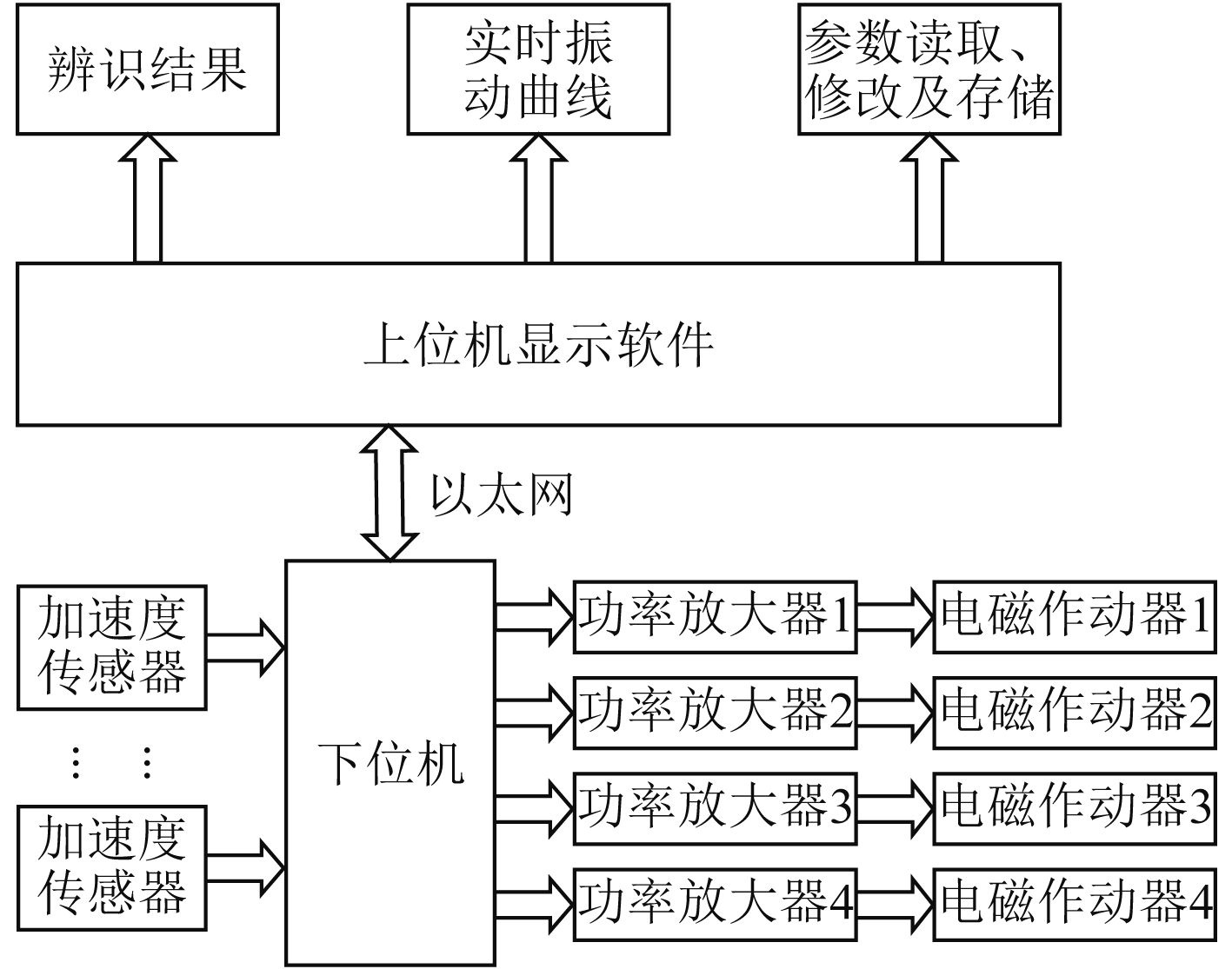图 1 主动隔振系统整体框架 Fig. 1 Active vibration isolation system overall frame
2 监控系统软件具体实现 2.1 通信设计图 2 自定义用户协议头结构 Fig. 2 Custom user protocol header structure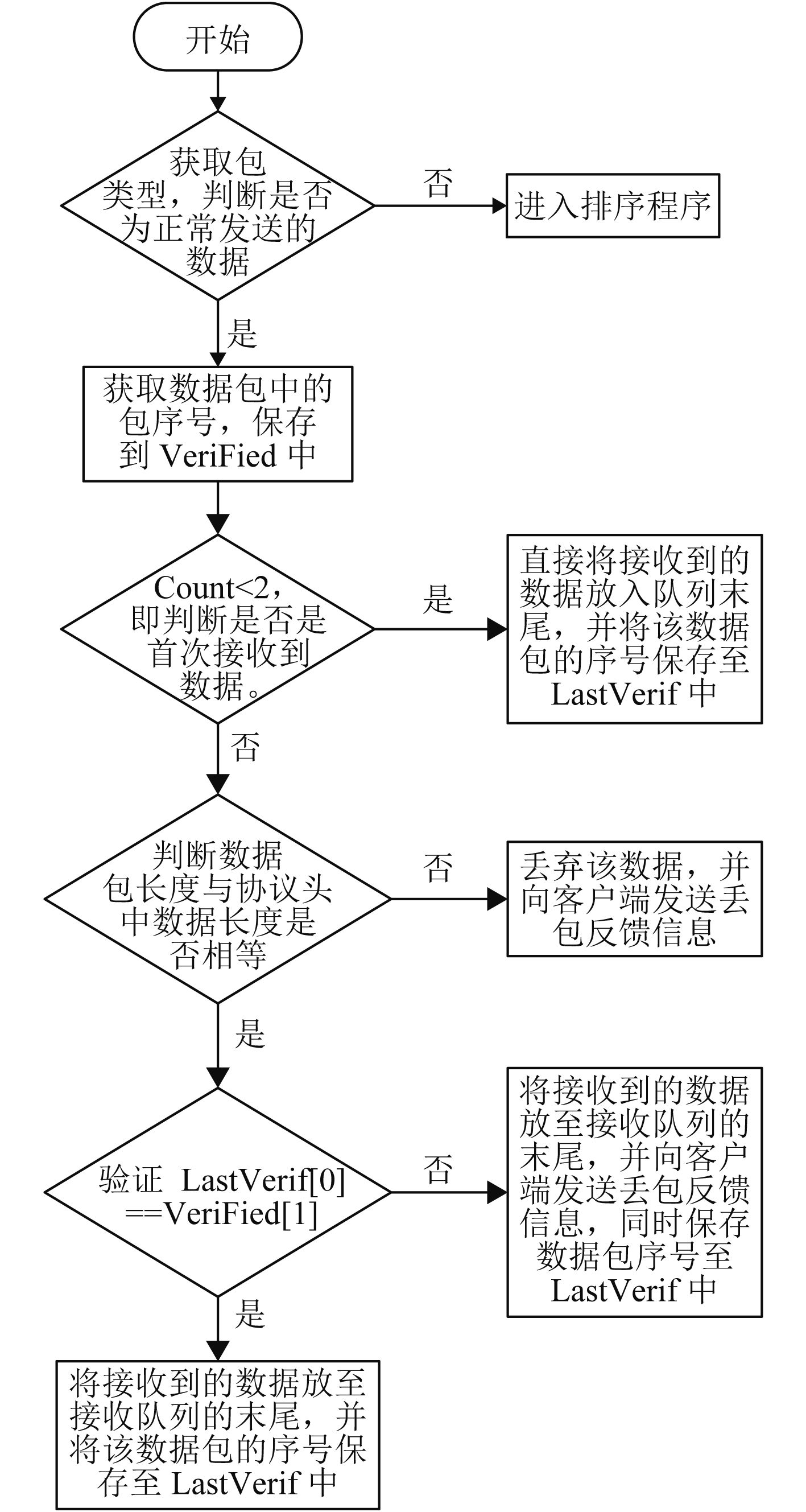图 3 客户端确认机制流程图 Fig. 3 Client confirmation mechanism flow chart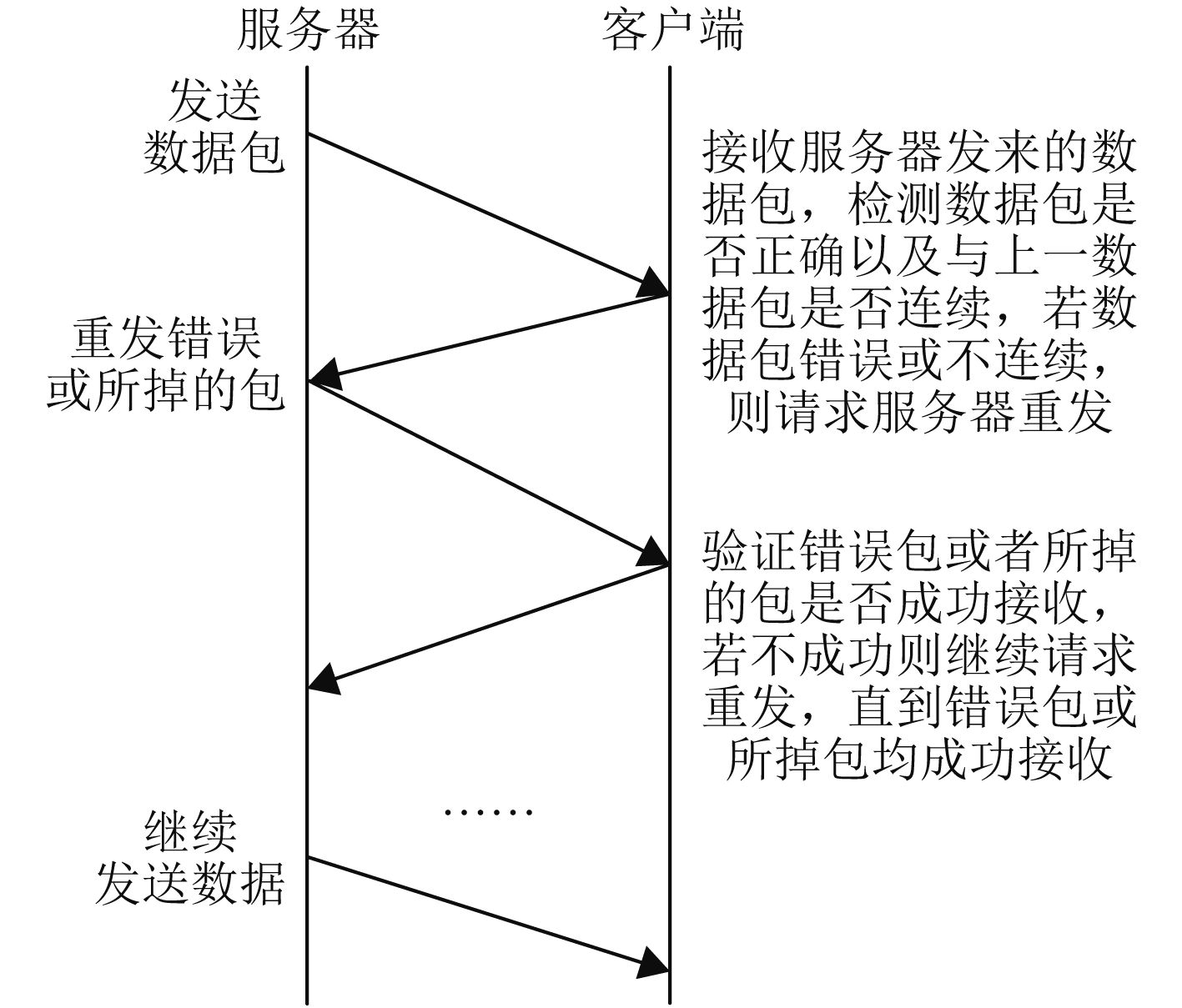图 4 重发机制流程图 Fig. 4 Retransmission mechanism flow chart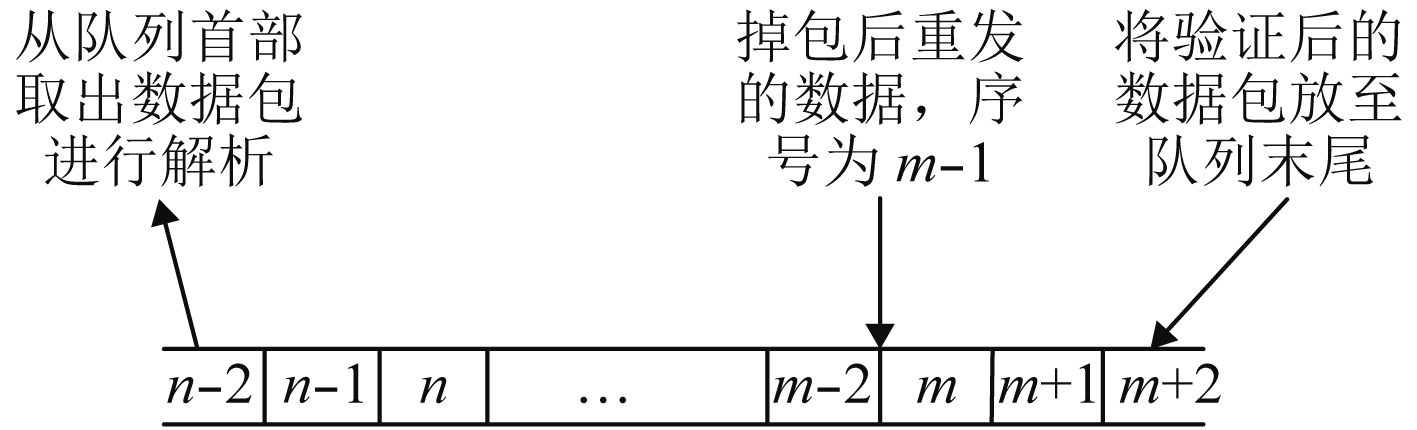图 5 客户端接收队列 Fig. 5 Client receive queue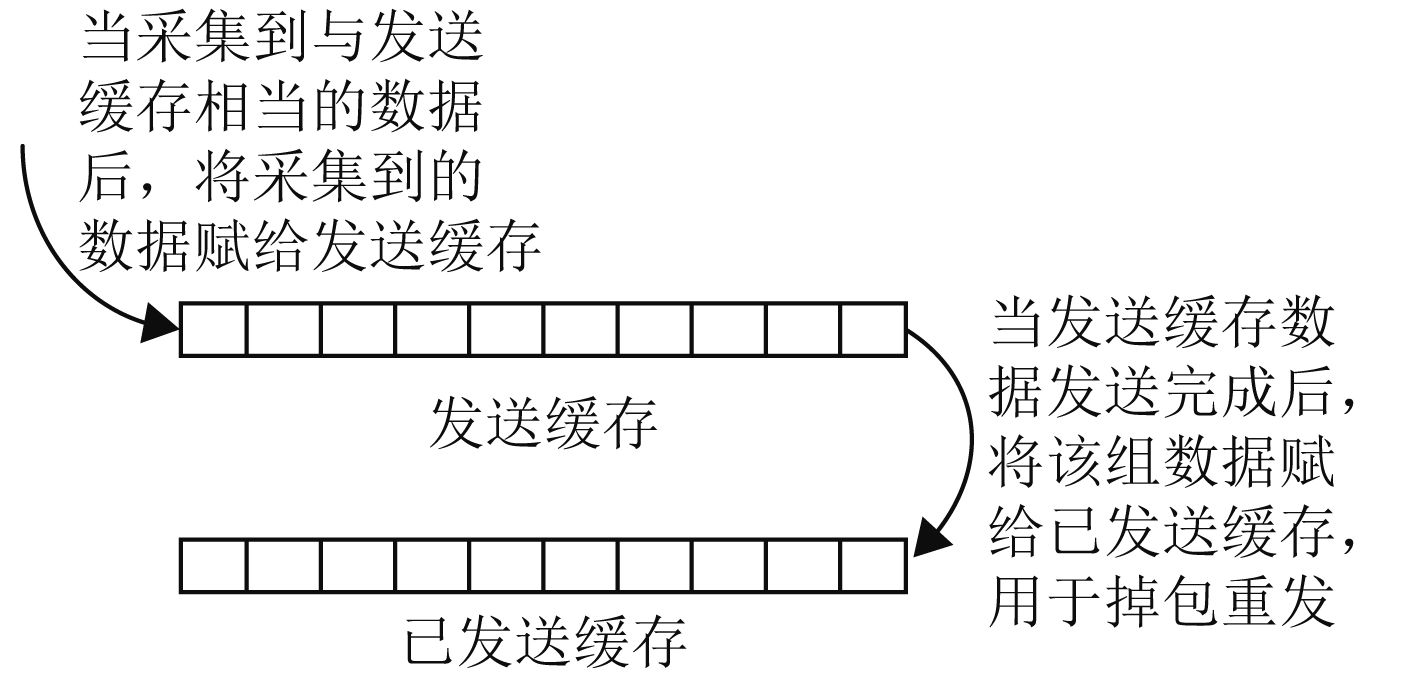图 6 服务器缓存区工作模式图 Fig. 6 Server cache area working mode diagram

2.2 参数配置及储存

1）创建XML文件

2）读取XML文件

3）向XML文件中添加数据

2.3 程序界面设计

1）通道辨识界面，主要用于显示接收下位机通道辨识得到的滤波器系数，并以曲线的形式显示在界面上，并且软件提供了辨识系数保存至本地和本地辨识系数下载的功能。此外，界面上留有指定区域用以显示下位机的报警信息，当下位机出现报警时，会在界面上以红色标出。

2）实时曲线界面，用于显示各个通道的实时数据，并可选择将其中4个通道的数据以曲线的形式显示在界面上，并且软件提供了数据保存功能，可以一次保存1 000个数据点，用于进一步的数据分析。此外，该界面也留有指定的报警显示区域。

3）参数配置界面，用于完成下位机配置信息的下载。打开该界面时，软件会读取下位机当前的配置信息并显示，辨识滤波器系数也会被读取，并以曲线的形式显示在通道辨识界面上。此外，软件提供了配置信息保存至本地和本地配置信息下载功能。设计好的系统界面主要功能模块如图7所示。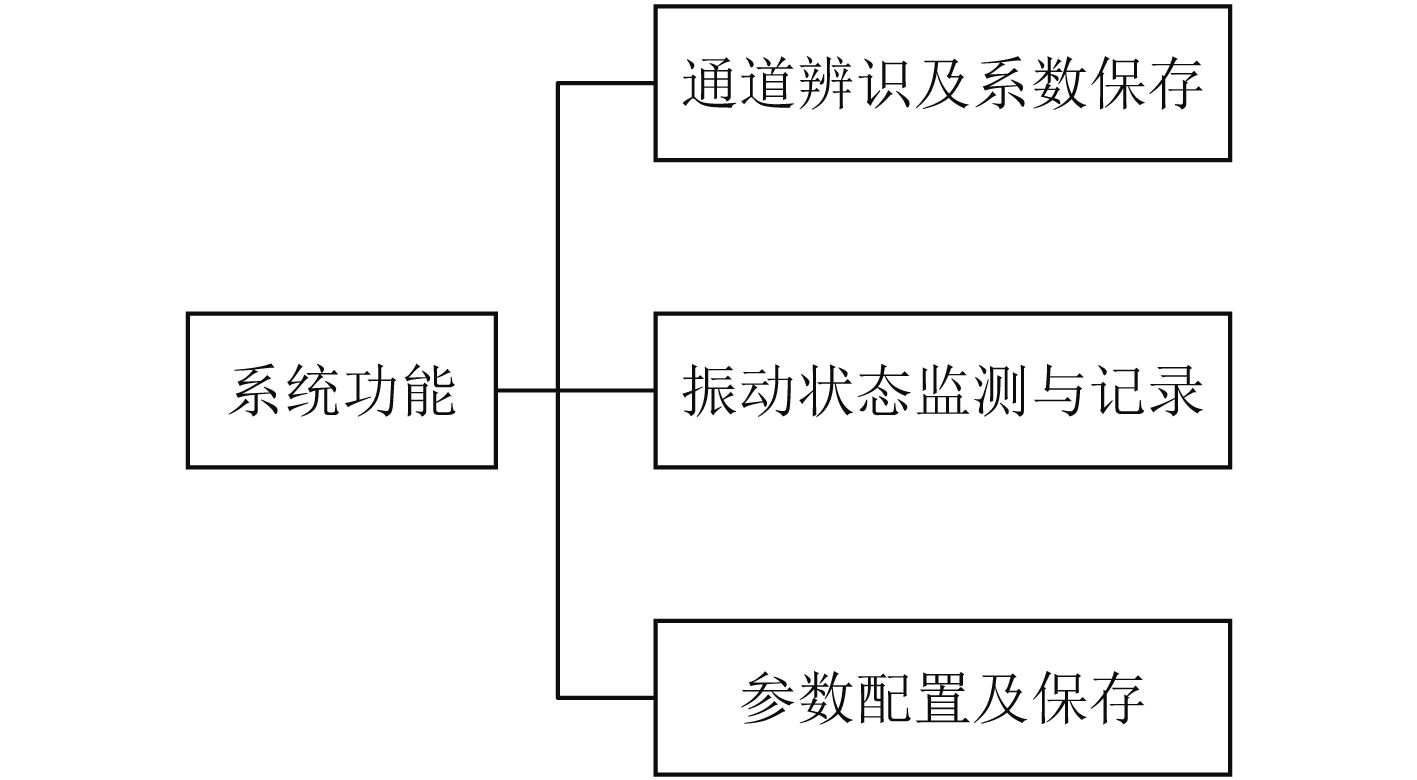图 7 系统界面功能模块图 Fig. 7 System interface function block diagram
3 自适应控制算法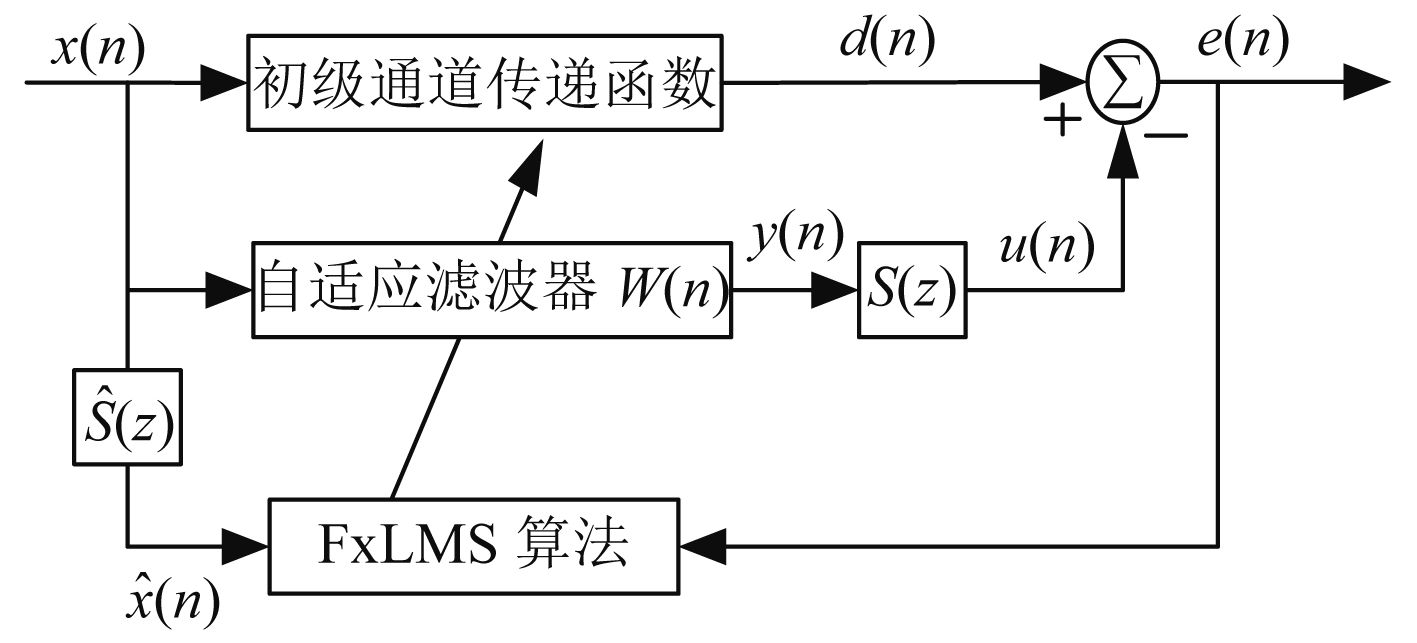图 8 次级通道估计模型计算流程图 Fig. 8 Secondary channel estimation model calculation flow chart

 $X\left( n \right)={{\left[ x\left( n \right),x\left( n-1 \right),\cdots ,x\left( n-L+1 \right) \right]}^{\rm T}}{\text{，}}$ (1)

 $W\left( n \right)={{\left[ {{w}_{0}},{{w}_{1}},\cdots ,{{w}_{L-1}} \right]}^{\rm T}}{\text{，}}$ (2)

 $S\left( n \right)={{\left[ {{s}_{0}},{{s}_{1}},\cdots ,{{s}_{M-1}} \right]}^{\rm T}}{\text{，}}$ (3)

 $e\left( n \right)=d\left( n \right)-u\left( n \right)=d\left( n \right)-S{{\left( n \right)}^{\rm T}}Y\left( n \right){\text{，}}$ (4)

 $Y\left( n \right)={{\left[ y\left( n \right),y\left( n-1 \right),\cdots ,y\left( n-L+1 \right) \right]}^{\rm T}}{\text{，}}$ (5)

 $e\left( n \right)=d\left( n \right)-\sum\nolimits_{l=0}^{L-1}{{{w}_{l}}\hat{x}\left( n-l \right)} {\text{。}}$ (6)

 $\hat{X}\left( n \right)={{\left[ \hat{x}\left( n \right),\hat{x}\left( n-1 \right),\cdots ,\hat{x}\left( n-L+1 \right) \right]}^{\rm T}}{\text{，}}$ (7)

 $e\left( n \right)=d\left( n \right)-{{\left[ \hat{X}\left( n \right) \right]}^{\rm T}}W\left( n \right){\text{，}}$ (8)

 $J\left( n \right)=E\left\{ {{e}^{2}}\left( n \right) \right\}{\text{，}}$ (9)

 $W\left( n+1 \right)=W\left( n \right)-\lambda \nabla J {\text{。}}$ (10)

 $\nabla J=\frac{\partial {{e}^{2}}\left( n \right)}{\partial w}=-2e\left( n \right)\hat{X}\left( n \right){\text{。}}$ (11)

 $W\left( n+1 \right)=W\left( n \right)+2\lambda e\left( n \right)\hat{x}\left( n \right){\text{。}}$ (12)

4 主动隔振系统验证 4.1 通信功能验证

4.2 主动隔振系统效果验证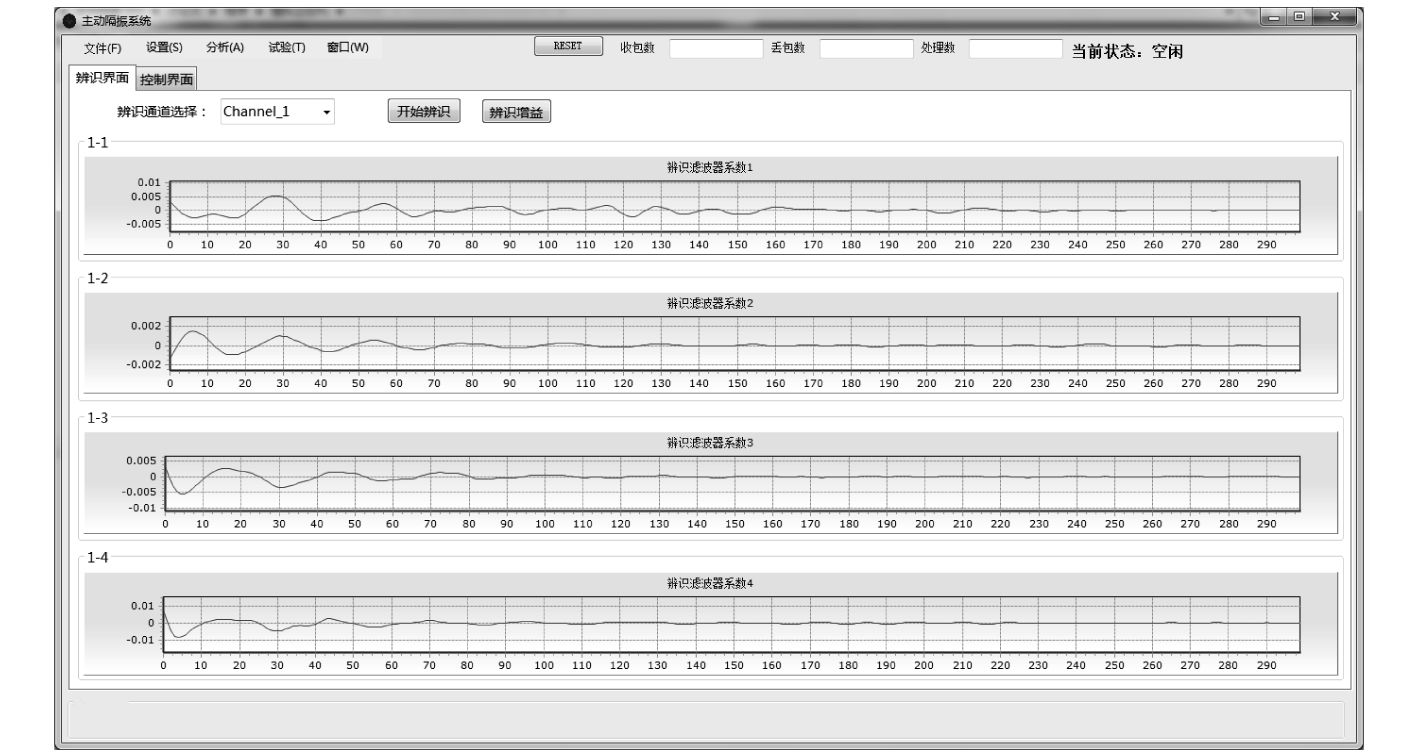图 9 次级通道辨识结果 Fig. 9 Secondary channel identification results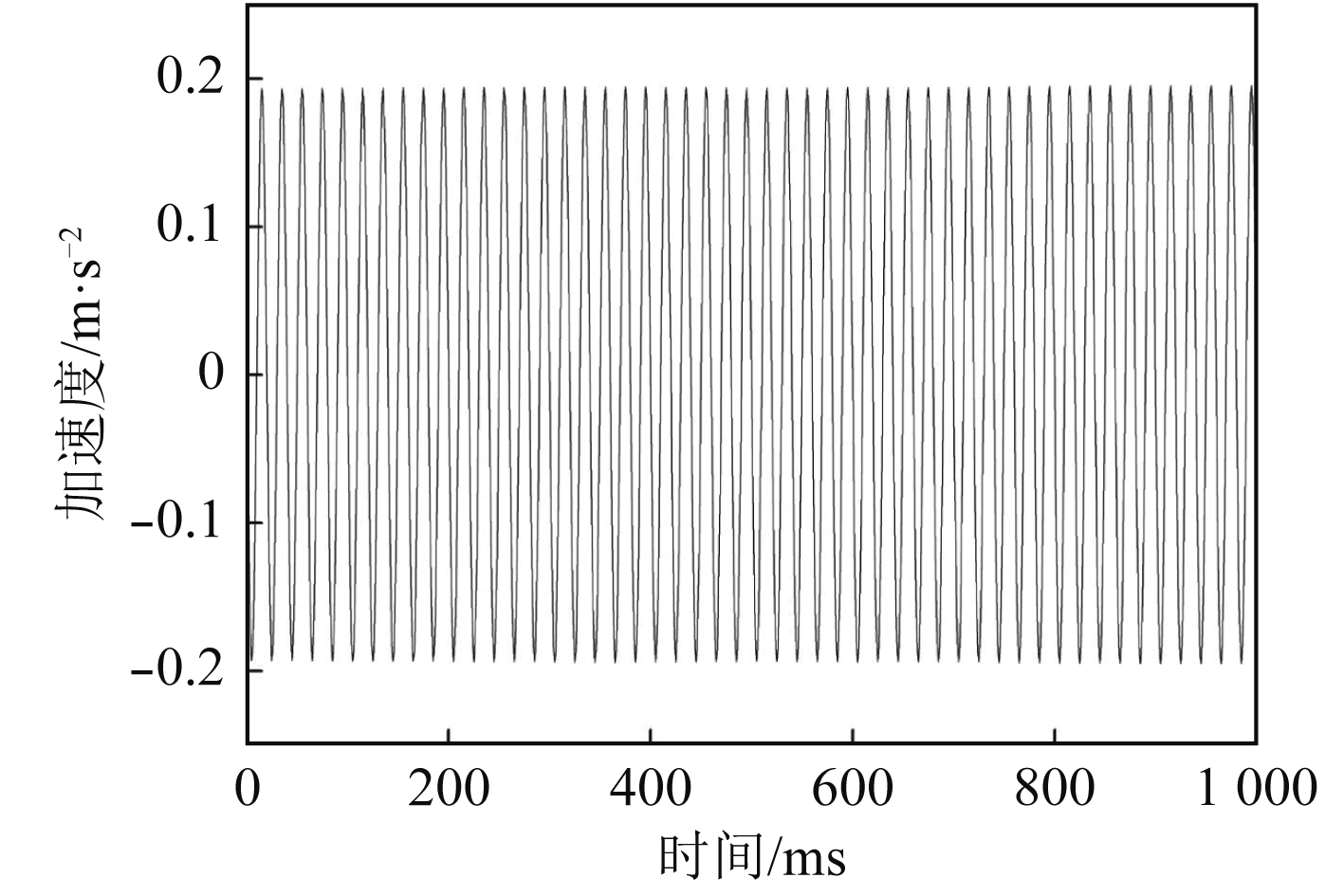图 10 控制数据 Fig. 10 Control data
5 结　语

  朱明刚. 船用柴油发电机组振动主动控制研究[D]. 哈尔滨: 哈尔滨工程大学, 2012.  JOEL Kanter. Understanding Thin-Client/Server Computing. ISBN 1-57231-744-2, 1997.  张攀. 船用往复式机械的振动主动控制技术研究[D]. 哈尔滨: 哈尔滨工程大学, 2013.  罗福强, 杨剑, 张敏辉. C#程序设计经典教程[M]. 北京出版社: 清华大学出版社, 2014  张锋. 基于压电作动器的齿轮传动系统振动主动控制及算法研究[D]. 重庆: 重庆大学, 2013.  张恺. 基于UDP的可靠文件传输协议的设计与实现[D]. 西安: 西安电子科技大学, 2014.